# 本文转载自看雪论坛【作者】rdsnow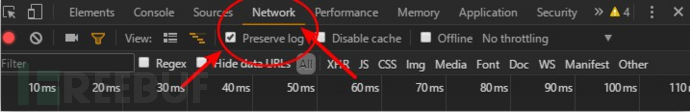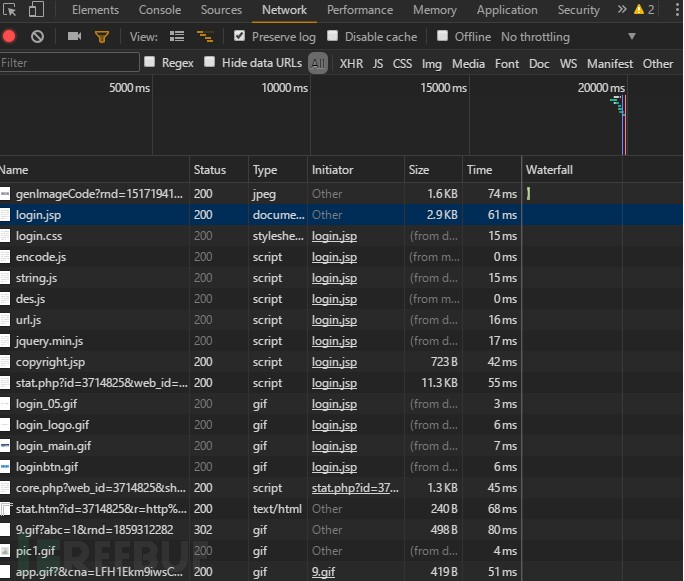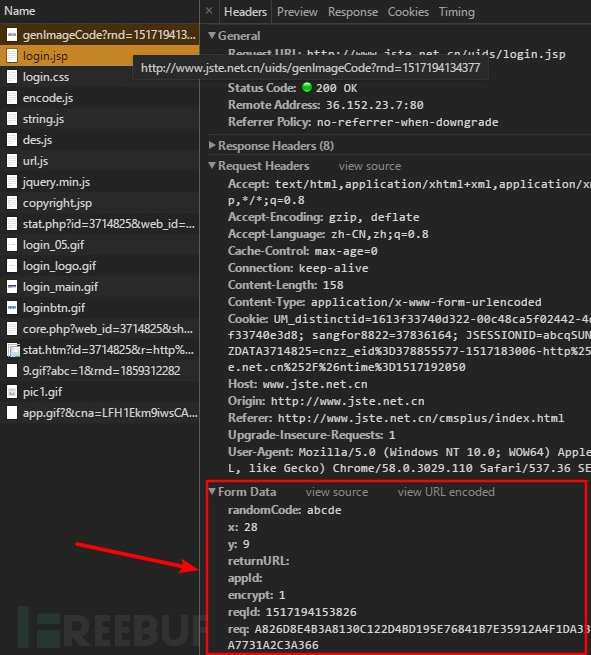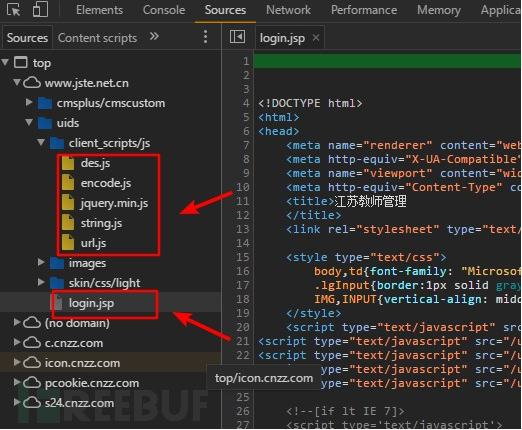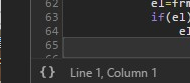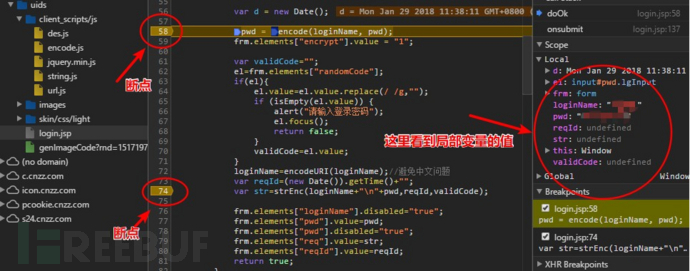F11单步进入第一个断点，这里需要点击界面下面的中括号重新格式化下代码，单步跟进后看到：

var _\$_7151 = ["encode", "ABCDEFGHIJKLMNOP", "QRSTUVWXYZabcdef", "ghijklmnopqrstuv", "wxyz0123456789+/", "=", "", "charCodeAt", "charAt", "length", "join", "reverse", "split"];window[_\$_7151] = function(c, e) {    function a(p) {        var q = _\$_7151 + _\$_7151 + _\$_7151 + _\$_7151 + _\$_7151;        p = encodeURI(p);        var r = _\$_7151;        var g, h, j = _\$_7151;        var k, l, m, o = _\$_7151;        var b = 0;        do {            g = p[_\$_7151](b++);//第一个字符            h = p[_\$_7151](b++);//第二个字符            j = p[_\$_7151](b++);//第三个字符            k = g >> 2; //得到 k            l = ((g & 3) << 4) | (h >> 4);//得到 i            m = ((h & 15) << 2) | (j >> 6);//得到 m            o = j & 63; //得到 o            if (isNaN(h)) {  //如果没有第二个字符                m = o = 64 //则取表中的第64个字符替换            } else {                if (isNaN(j)) { //如果没有第三个字符                    o = 64 //则取表中的第64个字符替换                }            }            ;r = r + q[_\$_7151](k) + q[_\$_7151](l) + q[_\$_7151](m) + q[_\$_7151](o);            g = h = j = _\$_7151;            k = l = m = o = _\$_7151        } while (b < p[_\$_7151]);;return r    }    var d = c[_\$_7151];    var f = a(e)[_\$_7151](_\$_7151)[_\$_7151]()[_\$_7151](_\$_7151);    for (var b = 0; b < (d % 2 == 0 ? 1 : 2); b++) {        f = a(f)[_\$_7151](_\$_7151)[_\$_7151]()[_\$_7151](_\$_7151)    }    ;return f}

function strEnc(data,firstKey,secondKey,thirdKey){    var leng = data.length;//取 data 的长度    var encData = “”;    var firstKeyBt,secondKeyBt,thirdKeyBt,firstLength,secondLength,thirdLength;    if(firstKey != null && firstKey != “”){        firstKeyBt = getKeyBytes(firstKey);//取 firstkey 在每个字符之间插入一个字节的 0        firstLength = firstKeyBt.length;//取得插入 0 后的长度    }    if(secondKey != null && secondKey != “”){        secondKeyBt = getKeyBytes(secondKey);//取 secondkey 在每个字符之间插入一个字节的 0        secondLength = secondKeyBt.length;//取得插入 0 后的长度    }    if(thirdKey != null && thirdKey != “”){ //登陆过程中，并没用到 thirdkey，即 thirdKey = None        thirdKeyBt = getKeyBytes(thirdKey);//取 thirdkey 在每个字符之间插入一个字节的 0        thirdLength = thirdKeyBt.length;//取得插入 0 后的长度    }    if(leng > 0){         if(leng < 4){ 如果 data 的长度＜4，因为跳过，代码用省略号替换            //省去一些代码……            }else{            var iterator = parseInt(leng/4);//data 的长度除 64，得到循环次数            var remainder = leng%4; //data 的长度是否是 64 位的整数倍，保存余数            var i=0;            for(i = 0;i < iterator;i++){ //开始循环                var tempData = data.substring(i*4+0,i*4+4); //循环取 data 的64 位                var tempByte = strToBt(tempData);//转换成 bits                var encByte ;                if(firstKey != null && firstKey !=“” && secondKey != null && secondKey != “” ){                    var tempBt;                    var x,y;                    tempBt = tempByte;                    for(x = 0;x < firstLength ;x ++){                        tempBt = enc(tempBt,firstKeyBt[x]);//循环从firstkey 中取得64 位做密钥，依次对 data 中的某一段加密                    }                    for(y = 0;y < secondLength ;y ++){                        tempBt = enc(tempBt,secondKeyBt[y]);//循环从second中取得64 位做密钥，依次对 data 中的某一段加密                    }                    encByte = tempBt;//保存加密结果                }            //…………            if(remainder > 0){  //如果 data 有多余的长度，不足64 位                var remainderData = data.substring(iterator*4+0,leng);                var tempByte = strToBt(remainderData);//将余下的分到4个16位的数组中                var encByte ;                if(firstKey != null && firstKey !=“” && secondKey != null && secondKey != “” && thirdKey != null ){                    var tempBt;                    var x,y,z;                    tempBt = tempByte;                    for(x = 0;x < firstLength ;x ++){                        tempBt = enc(tempBt,firstKeyBt[x]);循环从firstkey 中取得64 位做密钥，依次对 data 中的某一段加密                    }                    for(y = 0;y < secondLength ;y ++){                        tempBt = enc(tempBt,secondKeyBt[y]);循环从secondkey中取得64 位做密钥，依次对 data 中的某一段加密                    }                    encByte = tempBt;//保存加密结果                }                encData += bt64ToHex(encByte);//将加密后的文本转为16进制文本            }        }    }    return encData;//返回加密结果}

Permutation and translation tables for DES        __pc1 = [56, 48, 40, 32, 24, 16, 8,               0, 57, 49, 41, 33, 25, 17,               9, 1, 58, 50, 42, 34, 26,               18, 10, 2, 59, 51, 43, 35,               62, 54, 46, 38, 30, 22, 14,               6, 61, 53, 45, 37, 29, 21,               13, 5, 60, 52, 44, 36, 28,               20, 12, 4, 27, 19, 11, 3               ]

var keys = generateKeys(keyByte);

function generateKeys(keyByte){    var key   = new Array(56);    var keys = new Array();    keys[ 0] = new Array();    keys[ 1] = new Array();    keys[ 2] = new Array();    keys[ 3] = new Array();    keys[ 4] = new Array();    keys[ 5] = new Array();    keys[ 6] = new Array();    keys[ 7] = new Array();    keys[ 8] = new Array();    keys[ 9] = new Array();    keys = new Array();    keys = new Array();    keys = new Array();    keys = new Array();    keys = new Array();    keys = new Array();    var loop = [1,1,2,2,2,2,2,2,1,2,2,2,2,2,2,1];//看到了循环移位的表，没看到置换表    for(i=0;i<7;i++){        for(j=0,k=7;j<8;j++,k–){            key[i*8+j]=keyByte[8*k+i];//用了这个循环生成 56 位         }    }//省略代码}

…………

from Des import *

__pc1 = [        56, 48, 40, 32, 24, 16, 8, 0,        57, 49, 41, 33, 25, 17, 9, 1,        58, 50, 42, 34, 26, 18, 10, 2,        59, 51, 43, 35, 27, 19, 11, 3,        60, 52, 44, 36, 28, 20, 12, 4,        61, 53, 45, 37, 29, 21, 13, 5,        62, 54, 46, 38, 30, 22, 14, 6    ]

from Des import *from urllib.parse import quotefrom time import time, sleepfrom PIL import Imageimport requestsimport sysfrom bs4 import BeautifulSoups = requests.session()headers = {    ‘Cache-Control’: ‘max-age=0′,    ‘Connection’: ‘keep-alive’,    ‘Referer’: ,    ‘User-Agent’: ‘Mozilla/5.0 (Windows NT 10.0; WOW64) AppleWebKit/537.36 (KHTML, like Gecko) \    Chrome/58.0.3029.110 Safari/537.36 SE 2.X MetaSr 1.0′}def custom_encode(data):  # 懒得注释了，直接从js中拷贝出来，改成python的代码    tab = ‘ABCDEFGHIJKLMNOPQRSTUVWXYZabcdefghijklmnopqrstuvwxyz0123456789+/=’    data_bytes = list(data.encode())    while len(data_bytes) % 3 != 0:        data_bytes.append(0)    b = 0    length = len(data_bytes)    r =     while b < length:        g = data_bytes[b]        h = data_bytes[b + 1]        j = data_bytes[b + 2]        k = g >> 2        m = ((g & 3) << 4) | (h >> 4)        n = ((h & 15) << 2) | (j >> 6)        o = j & 63        third_char = ‘=’ if h == 0 else tab[n]        fourth_char = ‘=’ if j == 0 else tab[o]        r = r + tab[k] + tab[m] + third_char + fourth_char        b = b + 3    return r[::-1]  # 反序输出def encode_pwd(str_name, str_pwd):    encoded_pwd = custom_encode(str_pwd)    encoded_pwd = custom_encode(encoded_pwd)  # 先连续对密码加密两次    if len(str_name) % 2 == 1:        encoded_pwd = custom_encode(encoded_pwd)  # 如果用户名长度是奇数，则再加密一次    return encoded_pwddef strenc(data, firstkey, secondkey):    bts_data = extend_to_16bits(data)  # 将data长度扩展成64位的倍数    bts_firstkey = extend_to_16bits(firstkey)  # 将 first_key 长度扩展成64位的倍数    bts_secondkey = extend_to_16bits(secondkey)  # 将 second_key 长度扩展成64位的倍数    i = 0    bts_result = []    while i < len(bts_data):        bts_temp = bts_data[i:i + 8]  # 将data分成每64位一段，分段加密        j, k = 0, 0        while j < len(bts_firstkey):            des_k = des(bts_firstkey[j: j + 8], ECB)  # 分别取出 first_key 的64位作为密钥            bts_temp = list(des_k.encrypt(bts_temp))            j += 8        while k < len(bts_secondkey):            des_k = des(bts_secondkey[k:k + 8], ECB)  # 分别取出 second_key 的64位作为密钥            bts_temp = list(des_k.encrypt(bts_temp))            k += 8        bts_result.extend(bts_temp)        i += 8    str_result =     for each in bts_result:        str_result += ‘%02X’ % each  # 分别加密data的各段，串联成字符串    return str_resultdef extend_to_16bits(data):  # 将字符串的每个字符前插入 0，变成16位，并在后面补0，使其长度是64位整数倍    bts = data.encode()    filled_bts = []    for each in bts:        filled_bts.extend([0, each])  # 每个字符前插入 0    while len(filled_bts) % 8 != 0:  # 长度扩展到8的倍数        filled_bts.append(0)  # 不是8的倍数，后面添加0，便于DES加密时分组    return filled_btsdef get_rand_code():    random_code_url =     time_stamp = str(int(time() * 1000))    random_code_url += time_stamp    try:        req = s.get(random_code_url, headers=headers, stream=True)        with open(‘rand_code.jpg’, ‘wb’) as f:            for chunk in req.iter_content(chunk_size=1024):                f.write(chunk)    except requests.RequestException:        print(‘网络链接错误，请稍后重试/(ㄒoㄒ)/~~’)        sys.exit()    with Image.open(‘rand_code.jpg’)as img:        img.show()def login_site(reqid, randomcode, reqkey):    post_data = {        ‘randomCode’: randomcode,        ‘returnURL’: None,        ‘appId’: ‘uids’,        ‘site’: None,        ‘encrypt’: 1,        ‘reqId’: reqid,        ‘req’: reqkey    }    try:        req = s.post(, headers=headers, data=post_data)        print(‘Status Code：%s’ % req.status_code)  # 不知道为什么浏览器上登陆成功返回的是302，这里返回200        if ‘Set-Cookie’ in req.headers.keys():  # 还好，看到response中出现Set-Cookie，就登陆成功了            return True        else:            return False    except requests.RequestException:        print(‘网络链接错误，请稍后重试/(ㄒoㄒ)/~~’)        return Falsedef main():    print(.center(100, ‘-’))    uname = input(‘请输入你的用户名：’)    pwd = input(‘请输入你的登陆密码：’)    get_rand_code()    secondkey = input(‘请输入看到的验证码：’)  # 取得验证码，作为second_key，提交数据时作为 randomCode 的值    firstkey = str(int(time() * 1000))  # 取得提交时的时间戳，作为first_key，提交数据时候作为 reqId 的值    crypt_pwd = encode_pwd(uname, pwd)  # 对输入的密码进行第一次加密    data = quote(uname) + ‘\n’ + crypt_pwd  # 用户名URI编码后和密码加密后的文本链接等待被DES加密    post_req = strenc(data, firstkey, secondkey)  # 主要是DES计算，作为 req 的值提交数据    if login_site(reqid=firstkey, randomcode=secondkey, reqkey=post_req) is True:        print(.center(100, ‘-’))        print(‘登陆成功，O(∩_∩)O哈哈~…’)        try:            req = s.get()  # 打开一个网页测试一下            soup = BeautifulSoup(req.text, ‘html5lib’)  # 网页为多框架，测试下访问TOP框架中的文本            print(soup.select(‘.b’).text.replace(‘\n’, ).replace(‘ ‘, ))        except requests.RequestException:            print(‘网络链接错误，请稍后重试/(ㄒoㄒ)/~~’)if __name__ == ‘__main__’:  # 启动程序    main()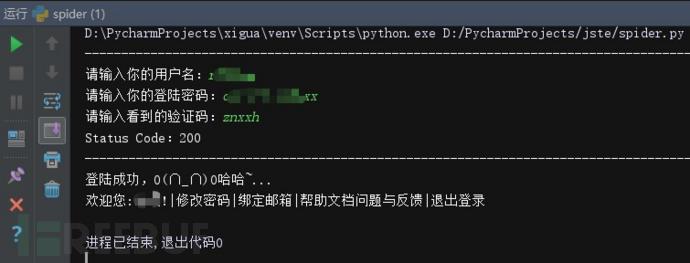firstkey 是提交时得到的时间戳，secondkey 就是输入的验证码